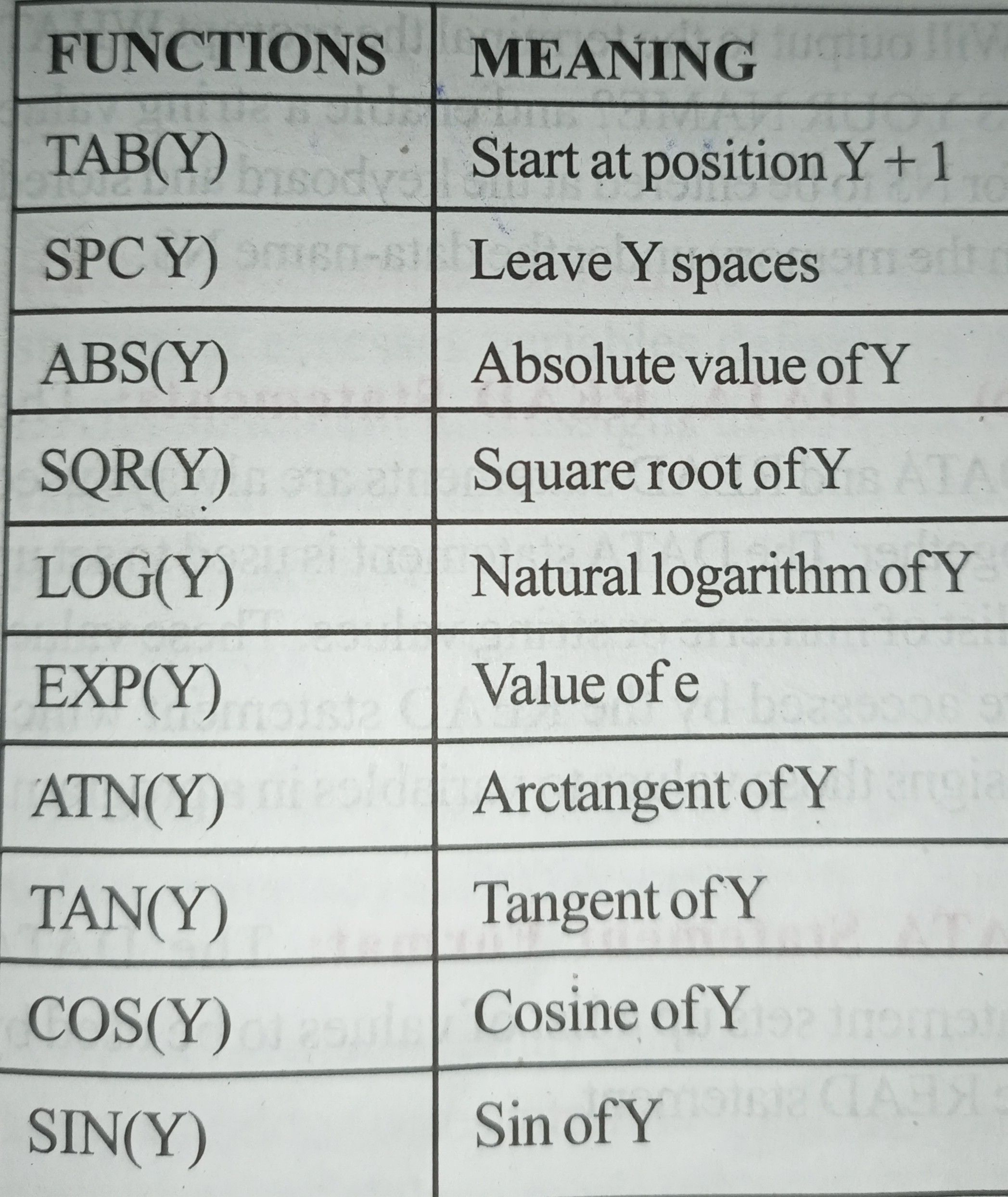Home » BASIC OPERATORS

# BASIC OPERATORSOperators perform mathematical or logical operations on values. The operators provided by BASIC are divided into four categories:

• Arithmetic.
• Relational.
• Logical.
• Functional.

(a) Arithmetic Operations: Operators BASIC performs addition, subtraction, multiplication, division and exponentiation, as well as more complicated operations.

The five operators used in writing most formulas are: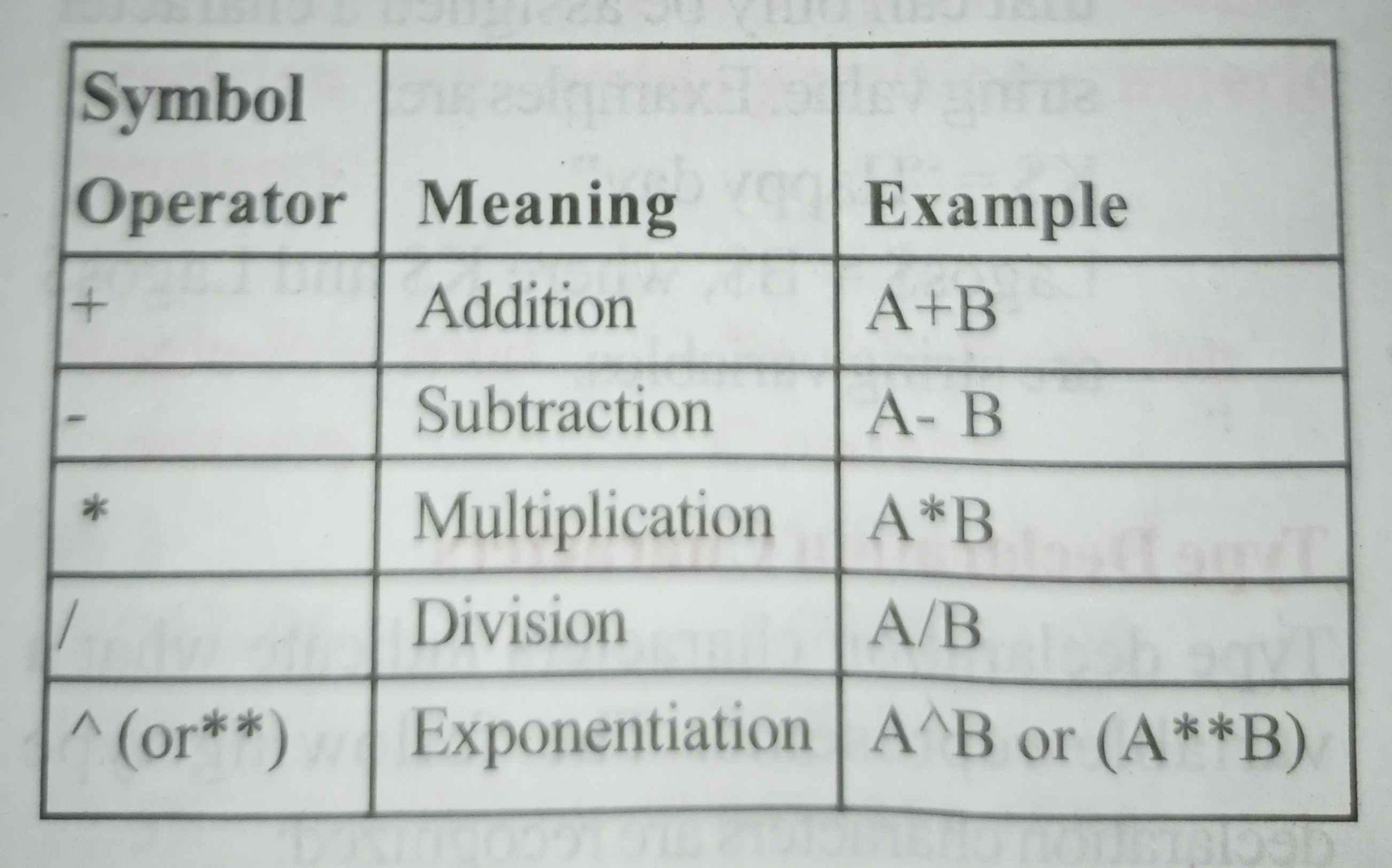(Raise A to the B Power)

Priority: In any given mathematical formula, BASIC performs the arithmetic operations in the following order:

i) Parentheses receive top priority. Any expression within parentheses is evaluated before an unparenthesized expression.

ii) In the absence of parentheses, the order or priority is:

• Exponentiation.
• Multiplication and Division (of equal priority).
• Addition and Subtraction (of equal priority).

(iii) If either sequence (i) or (ii) above does not clearly designate the order of priority, then the evaluation of expressions proceeds from left to right.

The expression A+B-C is evaluated from left to right as follows:

• A+B = step 1
• (Result of step 1) – C = answer

The expression A / B * C is also evaluated from left to right since multiplication and division are of equal priority:

• A/B = step 1
• (result of step 1)*C = answer

Parentheses – Parentheses may be used to change the order or priority because expressions within parenthesis are always evaluated first. Thus, by enclosing expressions appropriately, the order of evaluation can be controlled. Parentheses may be nested, that is, enclosed by one or more sets of parentheses. In this case, the expression within the innermost parentheses is evaluated first, and then the next innermost, and so on, until all have been evaluated. Consider the following example: A= 7*((B^2+4)/X)

The order of priority is:

1) B^2 = step 1

2) (result of step 1)+4 = step 2

3) (result of step 2)/X = step 3

4) (result of step 3)*7 = answer

(b) Relational Operation: A program may require that two values be compared at some point to discover their relation to one another. To accomplish this, BASIC makes use of the following relational operators: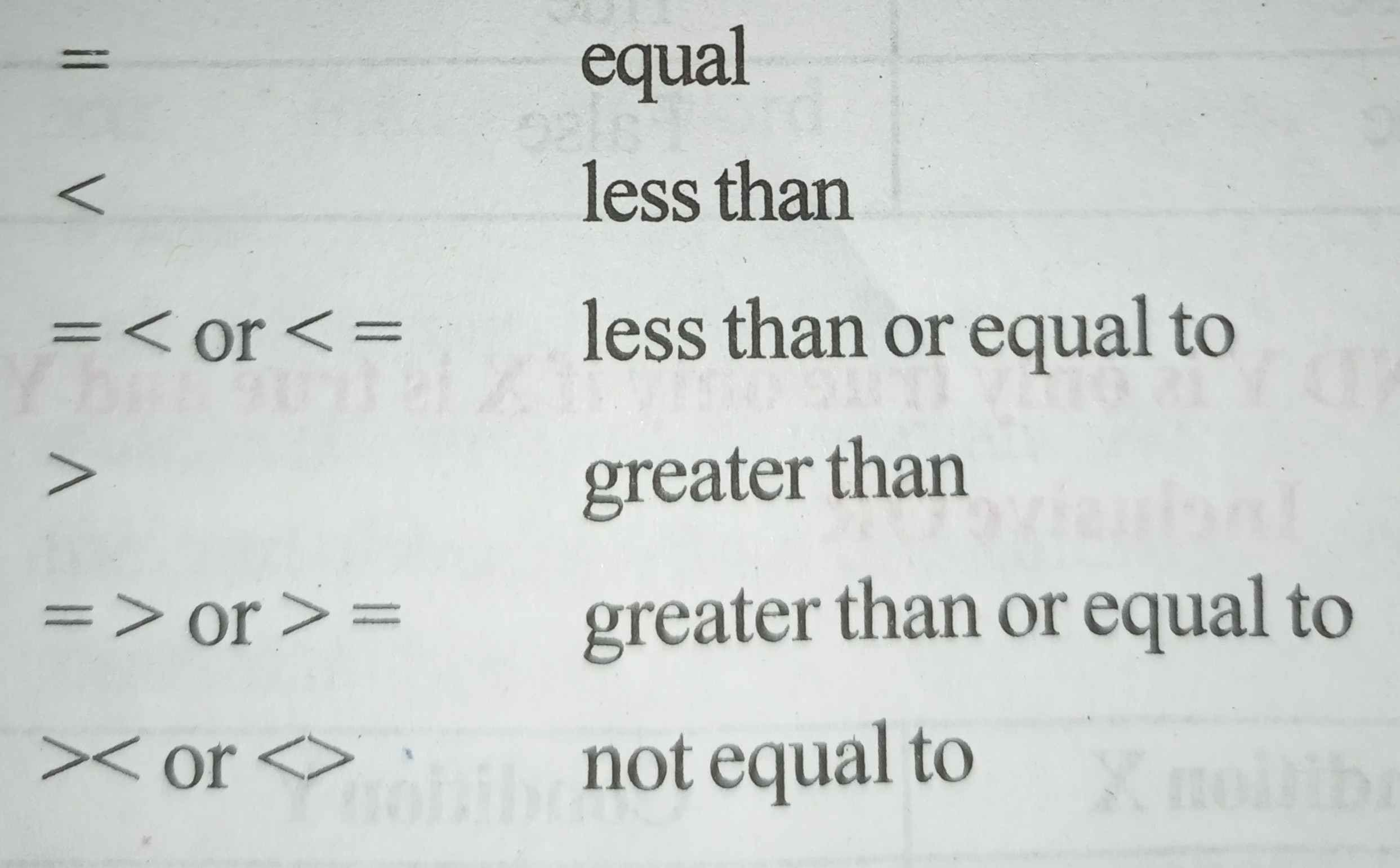Depending upon the result of the comparison, control of program execution may be directed to another part of the program. Relational operators are used in conjunction with the IF THEN statement.

When arithmetic and relational operators are combined in one expression, the arithmetic operator is always performed first.

(c) Logical Operation: Logical operators perform tests on multiple relations, bit manipulations, or Boolean operations, and return a true (not zero) or false (zero) value to be used in making decision. In an expression, logical operations are performed after arithmetic and relational operations. When a selection is based upon one or more conditions being true, it is possible to combine the condition together using logical operators and the resultant compound condition will either be true or false. The following truth tables illustrate the outcome of combining two conditions using the logical operators.

i) Logical AND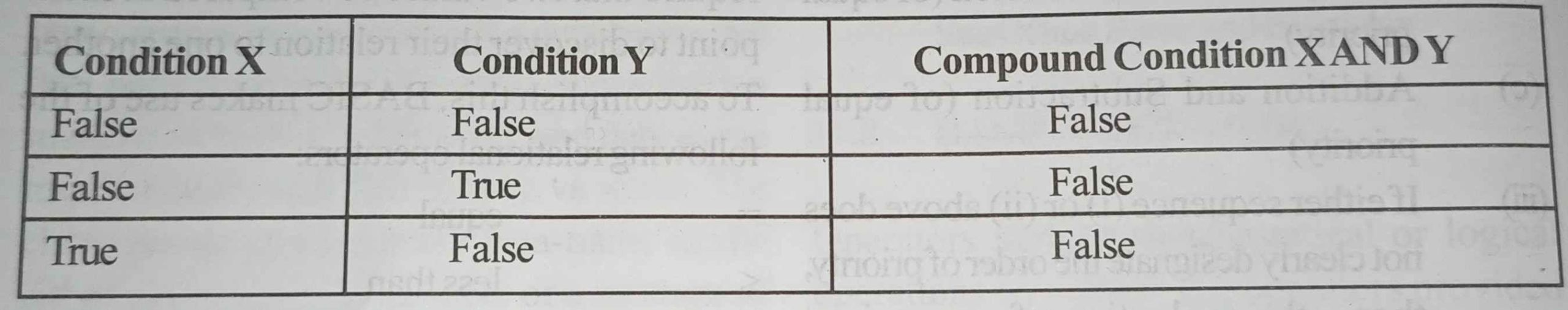X AND Y is only true only if X is true and Y is true.

i) Inclusive OR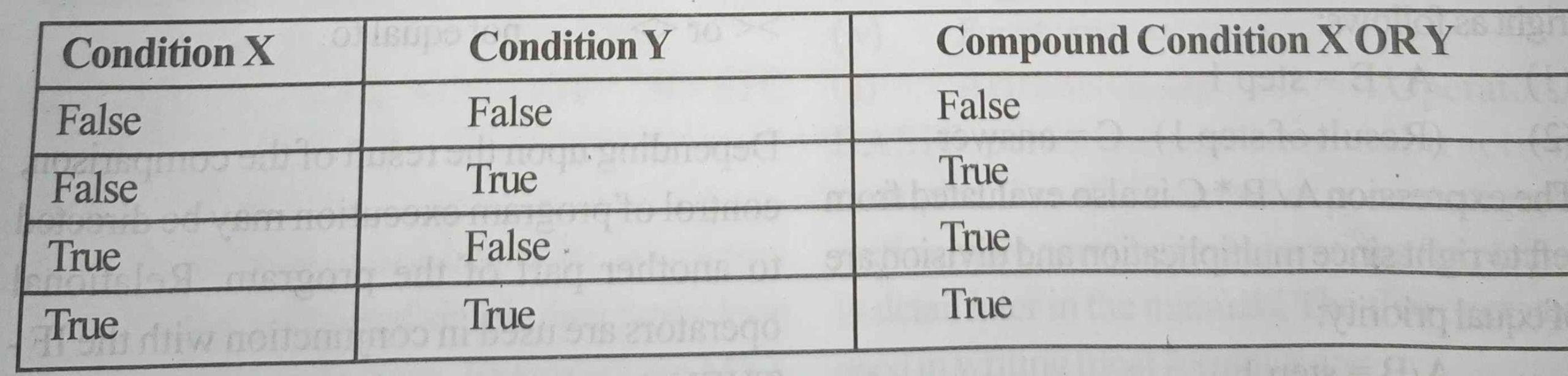X OR Y is only true only if X is true OR Y is true and including both being true.

i) Exclusive OR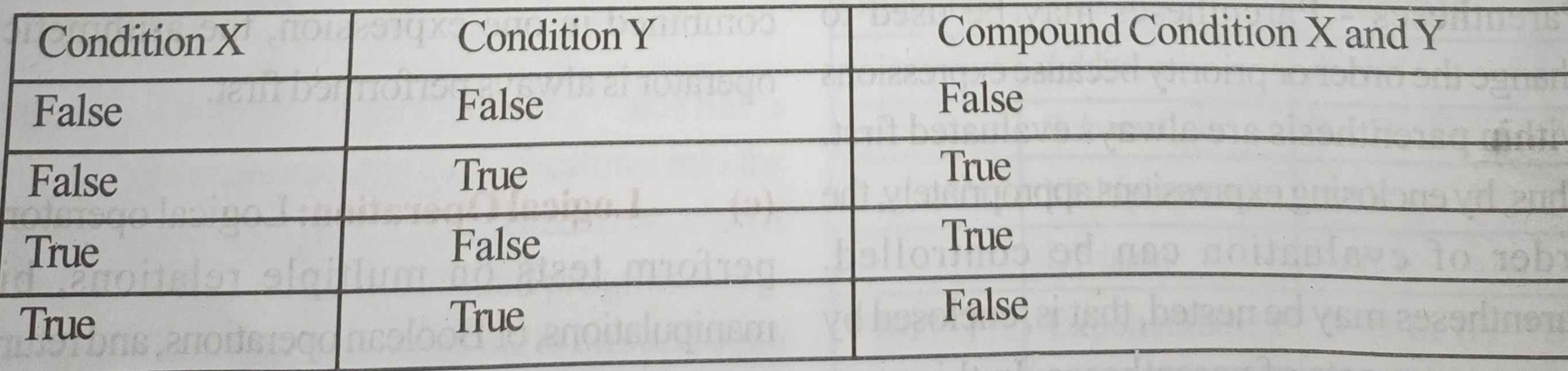X EOR Y is true only if X is true OR Y is true and excluding both being true and both being false.

(ii) NOT

The logical operator NOT negates or reverse a condition, so true become false and false becomes true.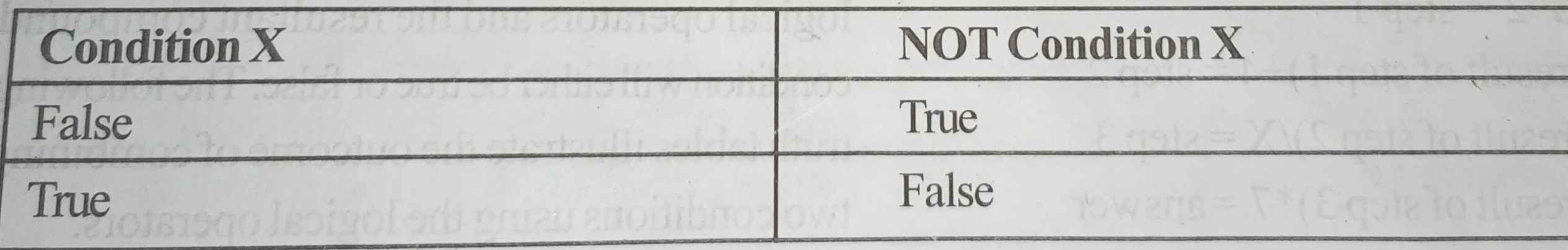There are six logical operators which are listed in order of precedence below: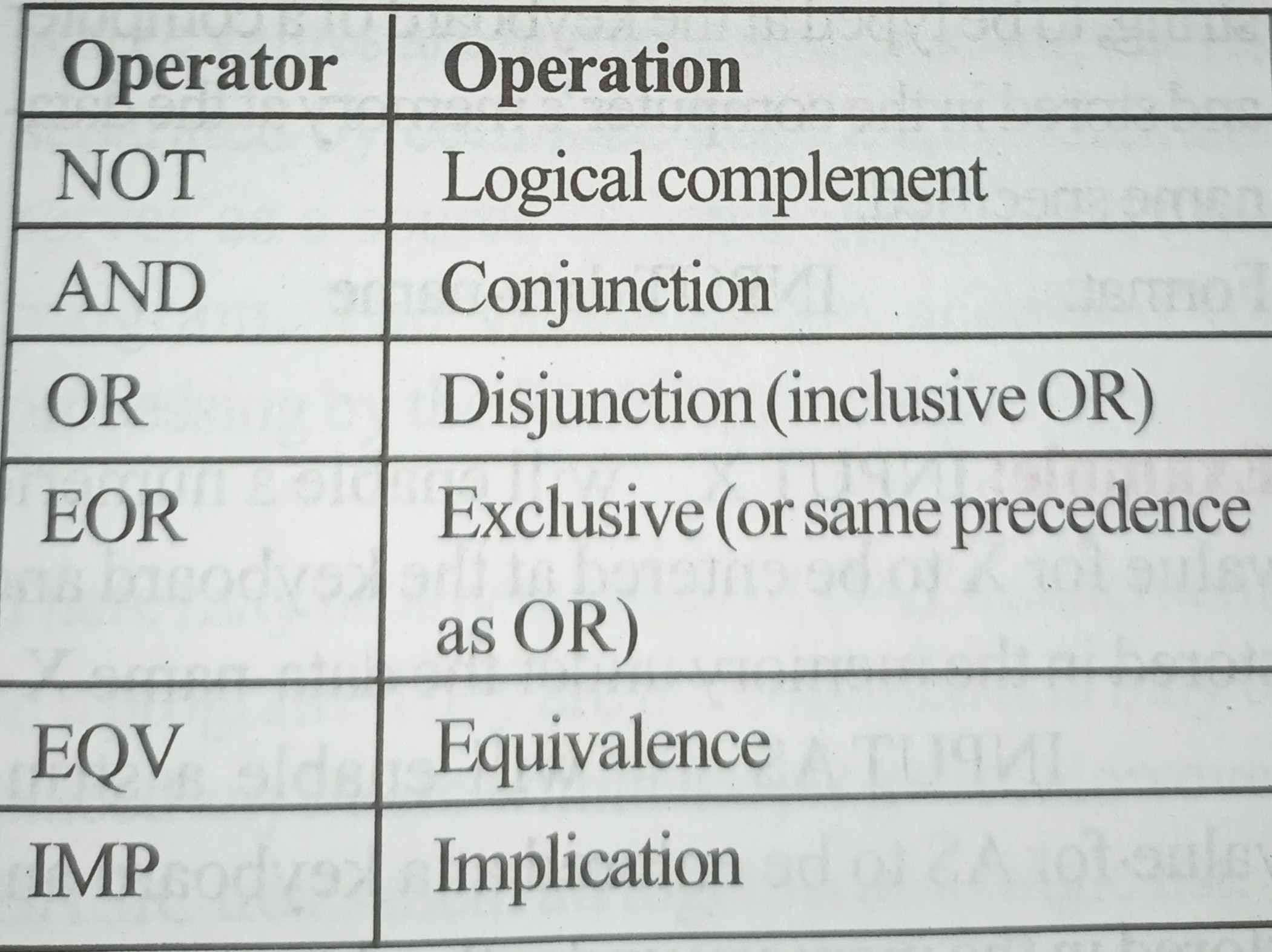(d) Functional Operators: A function is used in an expression to call a predetermined operation to be performed on an operand. Function calls consist of a three letter (all capitals) name followed by an argument in parenthesis. The argument may be a number, variable, expression, or another function. The functional operators and their meaning are given below: